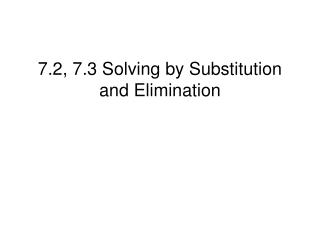DownloadDownload Presentation7.2, 7.3 Solving by Substitution and Elimination

# 7.2, 7.3 Solving by Substitution and Elimination

Télécharger la présentation## 7.2, 7.3 Solving by Substitution and Elimination

- - - - - - - - - - - - - - - - - - - - - - - - - - - E N D - - - - - - - - - - - - - - - - - - - - - - - - - - -
##### Presentation Transcript

1. 7.2, 7.3 Solving by Substitution and Elimination

2. Solving by Substitution 1) Use one equation to get either x or y by itself • Substitute what we just found in step 1 to the other equation • Solve for one unknown • Substitute the answer from step 3 to either of the original equation to solve for the other unknown • Check your answer

3. x + y = 4 x = y + 2 Step 1) x is already by itself x = y + 2 Step 2) Substitute to the first equation x + y = 4 (y + 2) + y = 4 Step 3) 2y + 2 = 4 2y = 4 – 2 = 2 y = 1 Step 4) x = y + 2 x = 1 + 2 = 3 Solution: (3, 1)

4. 2x + y = 6 3x + 4y = 4 Step 1) get y by itself 2x + y = 6 so y = -2x + 6 Step 2) Substitute to the second equation 3x + 4y = 4 3x + 4(-2x + 6) = 4 Step 3) 3x – 8x + 24 = 4 -5x + 24 = 4 -5x = -20 x = 4 Step 4) 2x + y = 6 2(4)+y = 6 so 8 + y = 6, y = -2 Solution: (4, -2)

5. More practice: (Make sure you check your answers) 1) 3x – 4y = 14 5x + y = 8 Answer: (2,-2) 2) 2x – 3y = 0 -4x + 3y = -1 Get x by itself: 2x – 3y = 0 so x = (3/2) y Substitute into: -4x + 3y = -1 -4(3/2)y + 3y = -1 -6y + 3y = -1 - 3y = -1 so y = 1/3 Solve for x: Use the first equation 2x – 3y = 0 2x – 3(1/3) = 0 2x – 1 = 0 x = ½ Answer: (1/2 , 1/3)

6. Example 1 • The perimeter of a basketball court is 288 ft. The length is 40 ft longer than the width. Find the dimensions of the court.

7. Example 2 • The sum of two numbers is 51. One number is 27 more than the other. Find the numbers.

8. Solve by Elimination • Multiply some numbers to either or both equations to get 2 opposite terms (For example: 2x and -2x) • Add equations to eliminate one variable • Solve for 1 unknown • Substitute the answer from step 3 to either of the original equation to solve for the other unknown • Check your answer

9. Solve by elimination 2x – 3y = 0 -4x + 3y = -1 Ignore step 1 since we already have 2 opposite terms -3y and 3y Step 2 &3: Add 2 equations to eliminate y and solve for x 2x – 3y = 0 -4x + 3y = -1 -2x = -1 x = 1/2 Step 4: Choose the first equation 2x – 3y = 0 2(1/2) – 3y = 0 1 - 3y = 0 - 3y = -1 y = 1/3 Answer: ( 1/2, 1/3)

10. Ex2) 2x + 2y = 2 3x – y = 1 Step 1: Multiply 2 to the second equation 2x + 2y = 2 (2) 3x – y = 1 2x + 2y = 2 6x – 2y = 2 8x = 4 (step 2 and 3) x = 1/2 Step 4: Choose 3x – y = 1 3(1/2) – y = 1 3/2 - y = 1 - y = 1 – (3/2) = - ½ y = ½ Answer: (1/2, 1/2)

11. Ex3) 2x + 3y = 8 -3x + 2y = 1 Step 1: Multiply 3 to the 1st equation and 2 to the 2nd equation (3) 2x + 3y = 8 (2) -3x + 2y = 1 6x + 9y = 24 -6x + 4y = 2 13y = 26 (step 2 and 3) y = 2 Step 4: Choose -3x + 2y = 1 -3x + 2(2) = 1 -3x + 4 = 1 -3x = 1 -4 = -3 x = 1 Answer: (1,2)

12. Practice Solve by elimination 1) 3x – 4y = 14 5x + y = 8 Answer: (2,-2) • 3x + 2y = 7 6x + 4y = 14 Answer: Infinite many solutions